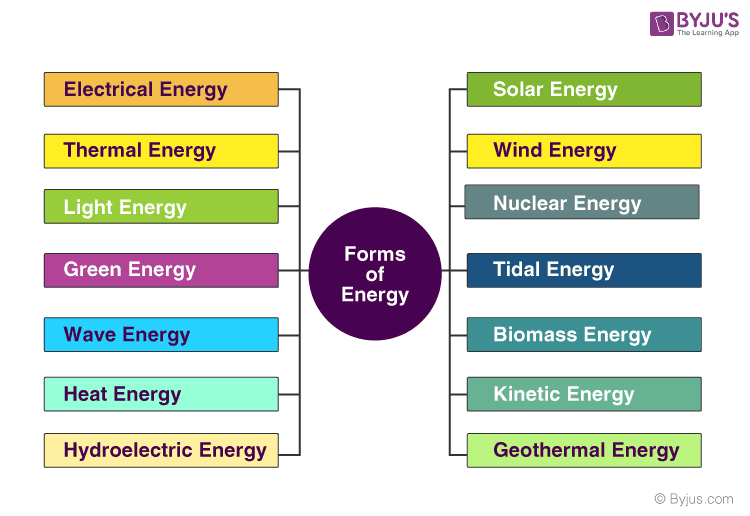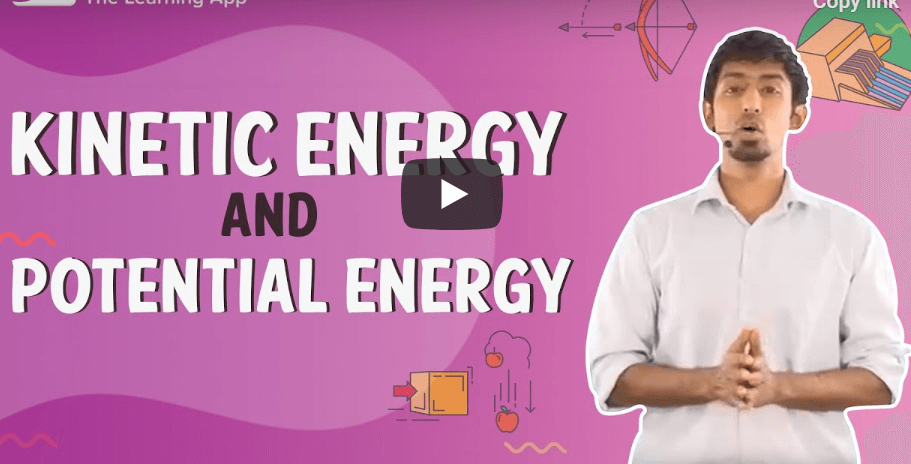# Energy and Classification Of Energy

## What is Energy

There are different forms of energy on this planet. The Sun is considered as the elemental form of energy on the Earth. In Physics, energy is considered a quantitative property which can be transferred from an object in order for it to perform work. Hence, we can define energy as the strength to do any kind of physical activity. Thus, they say,

Energy is the ability to do work

According to the laws of conservation of energy it states that “the energy can neither be created nor destroyed but can only be converted from one form to another”. The SI unit of energy is Joule. In this article, let us understand in detail about units of energy and different forms of energy.

### Units of Energy

The International System of Units of measurement of energy is Joule. The unit of energy is named after James Prescott Joule. Joule is a derived unit and it is equal to the energy expended in applying a force of one newton through a distance of one meter. However, energy is also expressed in many other units not part of the SI, such as ergs, calories, British Thermal Units, kilowatt-hours, and kilocalories, which require a conversion factor when expressed in SI units.

## Energy Conversion: Transfer and Transform

We know the energy can be transferred from one form to another, the movement of energy from one location to another is known as energy transfer. We notice various energy transformations happening around us.

Following are the four ways through which energy can be transferred:

• Mechanically – By the action of force
• Electrically – Electrically
• By Radiation – By Light waves or Sound waves
• By Heating – By conduction, convection, or radiation

The process which results in the energy changing from one form to another is known as energy transformation. While energy can be transformed or transferred, the total amount of energy does not change ­– this is called energy conservation.

### Law of Conservation of Energy

The law of conservation of energy is one of the basic laws in physics. It governs the microscopic motion of individual atoms in a chemical reaction. The law of conservation of energy states that “In a closed system, i.e., a system that is isolated from its surroundings, the total energy of the system is conserved.” According to the law, the total energy in a system is conserved even though the transformation of energy occurs. Energy can neither be created nor destroyed, it can only be converted from one form to another.

Read More: Law of Conservation of Energy

## Different Types of EnergyAlthough there are many forms of energy, it is broadly categorized into:

1. Kinetic Energy
2. Potential Energy

### Kinetic Energy

Kinetic energy is the energy associated with the object’s motion. Objects in motion are capable of causing a change or are capable of doing work. To better understand, let us think of a wrecking ball. A wrecking ball in motion is used to do work such as demolition of buildings, stones, etc. Even a slow-moving wrecking ball is capable of causing a lot of damage to another object such as an empty house. However, a wrecking ball that is not in motion, does not do any work.
Another example of kinetic energy is the energy associated with the constant, random bouncing of atoms or molecules. This is also known as thermal energy. The average thermal energy of a group of molecules is what we call temperature, and when thermal energy is being transferred between two objects, it’s known as heat.

 Kinetic energy is determined by the given formula $$\begin{array}{l}K.E=\frac{1}{2}mv^2\end{array}$$

#### Different Types of Kinetic Energy:

• Radiant energy refers to the type of energy that travels by waves or particles. This energy is created through electromagnetic waves and is most commonly experienced by humans in the form of heat. Following are a few examples of radiant energy:
• When you turn on an incandescent light bulb, it gives off two forms of energy. There is visible light and heat that is generated. Both these generated energies are a form of radiant energy.
• Sunlight is an example of radiant energy.
##### Thermal Energy

Thermal energy is similar to radiant energy and is experienced in the form of heat or warmth. While radiant energy refers to waves or particles, thermal energy describes the level of activity among the atoms and molecules in an object. This is the only difference between radiant energy and thermal energy. Some examples of thermal energy include:

• The geothermal energy that comes from the decay of natural minerals and the volcanic action of the earth is an example of thermal energy.
• When you heat up the pizza in the oven, you are raising the temperature of the pizza. The molecules that make up the pizza are moving more quickly when the pizza is piping hot.
• The warmth that you feel emanating from the engine is an example of thermal energy.
##### Sound Energy

The vibrations that reach the human ear are experienced by humans as sound. The disturbance moves in the form of waves through a medium like air and reaches our eardrum. On reaching the eardrum, these vibrations are converted into electrical signals and sent to the brain which we interpret as the sensation of sound.

##### Electrical Energy

The flow of negatively charged electrons around a circuit results in electricity which we more commonly referred to as electrical energy.

##### Mechanical Energy

Mechanical energy is the energy associated with the mechanical movement of objects.

### Potential Energy

Potential energy is the energy stored in an object or system of objects. Potential energy has the ability to transform into a more obvious form of kinetic energy.

 Potential energy is determined by the given formula $$\begin{array}{l}Potential\,Energy=m\times g\times h\end{array}$$

Both potential energy and kinetic energy form mechanical energy.

 Mechanical energy is determined by the following formula $$\begin{array}{l}Mechanical\,Energy=\frac{1}{2}mv^2+mgh\end{array}$$

#### Different Types Of Potential Energy

##### Gravitational Potential Energy

Gravitational potential energy is the energy stored in an object as the result of its vertical position or height. A book on a high bookshelf has a higher gravitational potential energy than the book at the bottom bookshelf.

##### Elastic Potential Energy

Elastic potential energy is energy stored as a result of applying a force to deform an elastic object. The energy is stored until the force is removed and the object springs back to its original shape, doing work in the process. The deformation could involve compressing, stretching or twisting the object.

##### Chemical Potential Energy

Chemical potential energy is the energy stored in the chemical bonds of the substance. It is the energy that can be absorbed and released due to a change in the particle number of the given species.

##### Electric Potential Energy

Electric potential energy is the energy that is needed to move a charge against an electric field.
Some of the examples of electric potential energy include:

• An incandescent light bulb that is turned off
• A radio tower that is not working
• A black-light turned off
• A television before it is turned on

### Recommended video on Kinetic Energy and Potential EnergyStay tuned to BYJU’S and FALL in Love with Learning!

## Frequently Asked Questions – FAQs

### What happens to the total energy of the object falling freely towards the ground?

The energy remains constant.

### What happens to the energy of a body on which work is done?

The body gains more energy.

### What is the commercial unit of energy?

The commercial unit of energy is Kilowatt-hour.

### Can energy be stored?

Yes, energy can be stored. One efficient way to store energy is in the form of chemical energy in a battery. When connected in a circuit, energy stored in the battery is released to produce electricity.
Energy can also be stored in many other ways. Batteries, gasoline, natural gas, food, water towers, a wound-up alarm clock, a Thermos flask with hot water all stores of energy.

### Which of the following is the energy possessed by its position?

• Kinetic Energy
• Potential Energy
• Mechanical Energy
• Electrical Energy

1. Tuba zafar

This is amazing

2. Good one for me

3. Thanks i got a help to do my project

4. Prince Singh

Its very useful to me. Thanks!

5. Mohit Singh

This is amazing

6. Yamer Khan

So Good!
Thanks for being here to help us